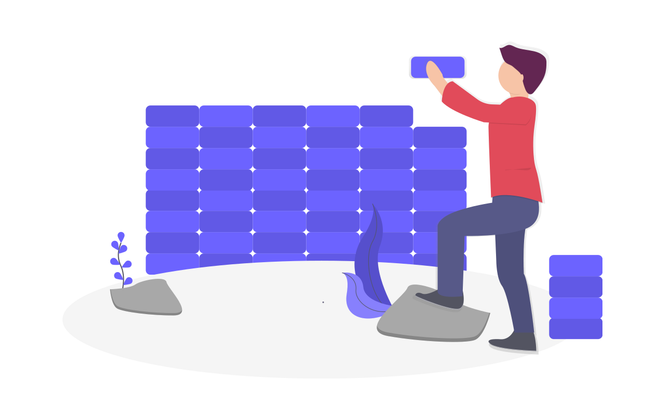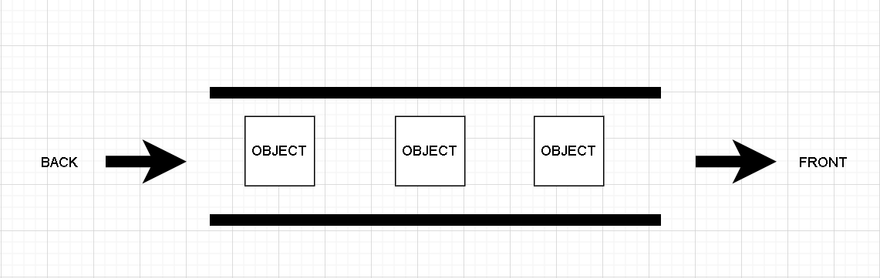## CodeNewbie Community is a community of 10,727 amazing newbies# Java Data Structures and Algorithms. Queues

### Introduction

• This series is going to be dedicated to learning and understanding data structures and algorithms in Java. All the information I am using is coming from the book, `Data Structures and Algorithms in Java by Michael T. Goodrich and Roberto Tamassia` and the California State Polytechnic University course, which is free and can be found HERE, `shout out professor Tang`. Please do not buy the book it is expensive and can be found online for MUCH cheaper.

• YouTube version of this blog post can be found HERE

### GitHub code

• GitHub for the code can be found HERE

### Queues

• Another fundamental data structure is the `Queue`, like a stack a queue is considered an abstract data type. The big difference between a stack and a queue is a queue is a collection of objects that are inserted and deleted according to the `first-in, first-out`(FIFO) principle. This means that elements can be inserted at any time, but only the element that has been in the queue the longest can be removed. We usually say that elements enter the queue from the `back` and are removed from the `front`. A common mental model to have when working with queues is a line up at the grocery store.### Methods

• The queue supports a total of 5 total methods:

1) enqueue(e) : Adds element e to the back of the queue

2) dequeue() : removes and returns the first element from the queue(or null if the queue is empty)

3 first() : returns the first element of the queue.

4) size() : returns the number of elements in the queue

5) isEmpty() : returns the number of elements in the queue

• Since a queue is an abstract data type we need to formalize these methods and we will do so with a Java interface.
``````public interface Queue <E>{

int size();

boolean isEmpty();

E first();

void enqueue(E e);

E dequeue();
}

``````
• Notice that with this interface we are using generics which allows more flexibility when defining types.

• For this queue data structure lets assume that elements are inserted into a array and the first element is at index 0, the second is at index 1 and so on. Which seems fairly obvious, however, things become a little less clear if we stop to think about how the `dequeue()` method would work. As we remove elements from the front of the queue, we have to keep updating the front, but how do we do the updating? The first solution that comes to mind is to remove whatever is in index 0 and then have a loop shift all other elements to the correct position. This solution does work but it runs in `O(N)` time and remember when dealing with data structure operations we want our method implementations to run in `O(1)` or `O(logN)` time. So, to optimize our run time and have it run in `O(1)` we will implement a circular array.

### Using Circular Arrays

• Basically a circular array is just a normal array but as we reach the end the array our index will wrap back around to the front of the array. To create a circular array all we have to do is implement one simple equation:

`f= (f+1)%N`

• Where `f` is used to represent the front of the queue and `N` is the length of the array. If you are unfamiliar with `%` it is called the `modulo operator` and will return the remainder of two operators. For example, if we have `10 % 10` the answer will be 0 and if we have `11 % 10` the answer will be 1. We will talk more about this equation later on.

### Implementing the queue

• To implement and create a queue we will follow 4 easy steps:

1) create the class and instance variables
2) create the constructors
3) implement the interfaces
4) implement the methods

### 1) Create the class and instance variables

• Our class will have 4 instance variables:

1) data : used to hold a reference to the array used for data storage

2) f : used to hold a reference to the front of the queue

3) size : used to determine how many elements are in our queue

4) CAPACITY : used as a default capacity value for our array. Technically, it this is a class variable and not an instance variable.

``````public class ArrayQueue <E>{
private E[] data;
private int f;
private int size = 0;
private static final int CAPACITY = 1000;
}
``````
• As you can see with `CAPACITY` the static modifier makes it a class variable and not an instance variable.

### 2) Create the constructors

• Now we will create the constructors, we will create two constructors to give our class a little bit of flexibility when it comes to array size.
``````public class ArrayQueue <E>{
//...
public ArrayQueue(){
this(CAPACITY);
}
public ArrayQueue(int capacity){
data = (E[]) new Object[capacity];
}
}
``````
• If the line `(E[]) new Object[capacity];` confuses you read THIS stack overflow for clarification. But essentially we have to do our little `(E[]) new Object[capacity];` hack because of `type erasures` and that fact we can not directly instantiate a type parameter.

### 3) implement the interfaces

• This step is very straight forward, all we do is implement the interface that we created earlier.
``````public class ArrayQueue <E> implements Queue<E> {
@Override
public int size() {
return 0;
}

@Override
public boolean isEmpty() {
return false;
}

@Override
public E first() {
return null;
}

@Override
public void push(E e) {

}

@Override
public E pop() {
return null;
}

}
``````

### 4) Implement the methods

• The first 3 methods, `size()`, `isEmpty()` and `first()` are the easiest to implement:
``````    @Override
public int size() {
return size;
}

@Override
public boolean isEmpty() {
return size == 0;
}

@Override
public E first() {
if(isEmpty()) return null;
return data[f];
}

``````
• The only method that might be slightly confusing is `first()` and all we have to remember is that `f` represents the front of the queue and will be shifting as we remove elements from the queue.

#### enqueue(E e)

• This is the first method that needs a little explaining:
``````    @Override
public void enqueue(E e) throws IllegalStateException{
if(size == data.length) throw new IllegalStateException("QUEUE IS FULL");
int back= (f +size) % data.length;
data[back] = e;
size++;
}
``````
• The goal of the enqueue method is to add a new element to the back of the queue. We need to determine the proper index at which to place the new element. Although, we do not explicitly maintain an reference variable for the back of the queue(like most other queue implementations do), we can compute the next opening with this equation:
`int back= (f +size) % data.length;`

• Then we simply assign the `e` element to its proper index `data[back] = e;` and increase the size of the queue `size++;`

#### dequeue()

• When this method is called the current value of `f` designates the index of the value that we want to remove and return. We keep a local reference to the element that will be returned, `answer`, before setting its cell of the array back to null. Then the index f is updated to reflect the removal of the first element, and the presumed promotion of the second element to become the new first. In most cases we would just increment the index by one, but because of the possibility of a wrap around configuration (circular array) we will rely on modular arithmetic the equation: `f = (f +1) % data.length;`
``````    @Override
public E dequeue() {
if(isEmpty()) return null;
f = (f +1) % data.length;
size--;

}
``````

### Analyzing the efficiency of an array based queue

• In our array based queue implementation each of the methods execute a fixed number of operations, thus making each method run in `O(1)` constant time(Which is what we want for data structure operations).

• The space usage is `O(N)`, where N is the size of the array, determined at the time the queue is created and independent from from the number of elements actually in the queue.

### Conclusion

• Thank you for taking the time out of your day to read this blog post of mine. If you have any questions or concerns please comment below or reach out to me on Twitter.